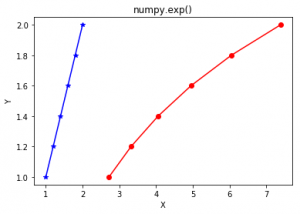Related Articles
numpy.exp() in Python
• Last Updated : 29 Nov, 2018

numpy.exp(array, out = None, where = True, casting = ‘same_kind’, order = ‘K’, dtype = None) :
This mathematical function helps user to calculate exponential of all the elements in the input array.

Parameters :

```array    : [array_like]Input array or object whose elements, we need to test.
out      : [ndarray, optional]Output array with same dimensions as Input array,
placed with result.
**kwargs : Allows you to pass keyword variable length of argument to a function.
It is used when we want to handle named argument in a function.
where    : [array_like, optional]True value means to calculate the universal
functions(ufunc) at that position, False value means to leave the
value in the output alone.
```

Return :

```An array with exponential of all elements of input array.
```

Code 1 : Working

 `# Python program explaining``# exp() function``import` `numpy as np`` ` `in_array ``=` `[``1``, ``3``, ``5``]``print` `(``"Input array : "``, in_array)`` ` `out_array ``=` `np.exp(in_array)``print` `(``"Output array : "``, out_array)`

Output :

```Input array :  [1, 3, 5]
Output array :  [   2.71828183   20.08553692  148.4131591 ]
```

Code 2 : Graphical representation

 `# Python program showing``# Graphical representation of ``# exp() function``import` `numpy as np``import` `matplotlib.pyplot as plt`` ` `in_array ``=` `[``1``, ``1.2``, ``1.4``, ``1.6``, ``1.8``, ``2``]``out_array ``=` `np.exp(in_array)`` ` `y ``=` `[``1``, ``1.2``, ``1.4``, ``1.6``, ``1.8``, ``2``]``plt.plot(in_array, y, color ``=` `'blue'``, marker ``=` `"*"``)`` ` `# red for numpy.exp()``plt.plot(out_array, y, color ``=` `'red'``, marker ``=` `"o"``)``plt.title(``"numpy.exp()"``)``plt.xlabel(``"X"``)``plt.ylabel(``"Y"``)``plt.show()  `

Output :Attention geek! Strengthen your foundations with the Python Programming Foundation Course and learn the basics.

To begin with, your interview preparations Enhance your Data Structures concepts with the Python DS Course.

My Personal Notes arrow_drop_up